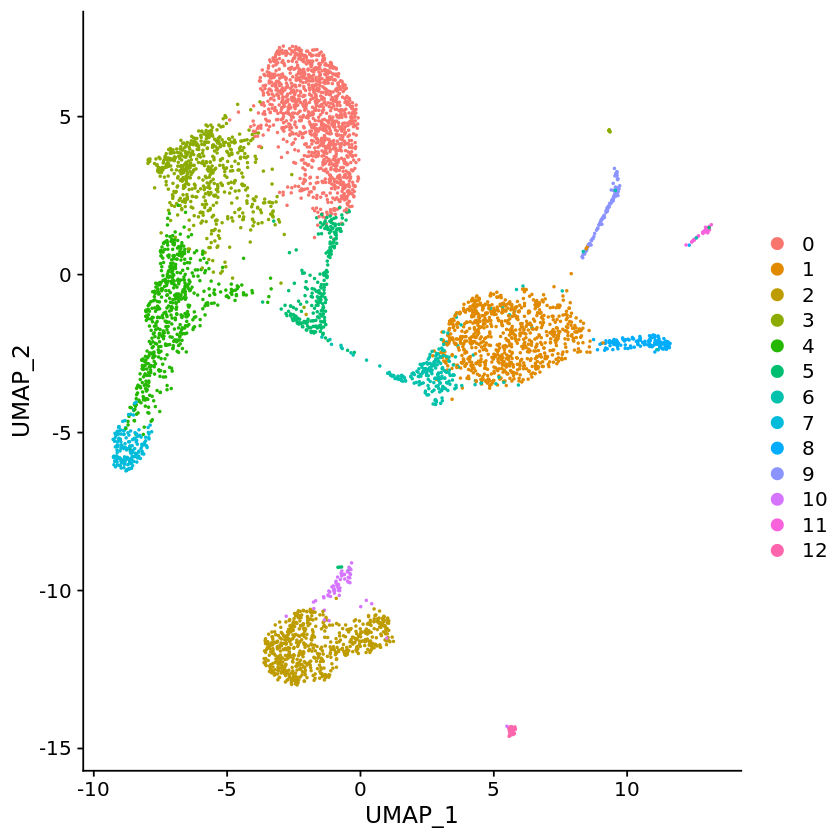## Alevin-Seurat Connection

``````# Seurat v3.0.0 and tximport >=1.13.10
library(Seurat)
library(tximport)
``````
``````# path to the output directory of Alevin run of pbmc_1k 10x V2 data
files <- file.path("alevin_quants/alevin/quants_mat.gz")
file.exists(files)
``````
``````"TRUE"
``````
``````# Reading in the alevin quants quants
txi <- tximport(files, type="alevin")
``````
``````"and we are good to go !! Cells after this has been taken from Seurat tutorial:
https://satijalab.org/seurat/v3.0/pbmc3k_tutorial.html
Below lines are for example purposes only and could be suboptimal. We recommend
checking out Seurat tool for more detailed tutorial of the downstream analysis."
``````
``````pbmc <- CreateSeuratObject(counts = txi\$counts , min.cells = 3, min.features = 200, project = "10X_PBMC")
``````
``````pbmc <- NormalizeData(pbmc, normalization.method = "LogNormalize", scale.factor = 10000)
``````
``````pbmc <- FindVariableFeatures(pbmc, selection.method = "vst", nfeatures = 2000)

all.genes <- rownames(pbmc)
pbmc <- ScaleData(pbmc, features = all.genes)
``````
``````pbmc <- RunPCA(pbmc, features = VariableFeatures(object = pbmc))
``````
``````pbmc <- FindNeighbors(pbmc, dims = 1:10)
pbmc <- FindClusters(pbmc, resolution = 0.5)
``````
``````pbmc <- RunUMAP(pbmc, dims = 1:10)
``````
``````DimPlot(pbmc, reduction = "umap")
``````